# EMS - Autobahn you Media: Music, Videos, eBooks, Audiobooks!function(t,n){"object"==typeof exports&&"undefined"!=typeof module?module.exports=n():"function"==typeof define&&define.amd?define(n):(t="undefined"!=typeof globalThis?globalThis:t||self).__SVGATOR_PLAYER__=n()}(this,(function(){"use strict";function t(n){return(t="function"==typeof Symbol&&"symbol"==typeof Symbol.iterator?function(t){return typeof t}:function(t){return t&&"function"==typeof Symbol&&t.constructor===Symbol&&t!==Symbol.prototype?"symbol":typeof t})(n)}function n(t,n){if(!(t instanceof n))throw new TypeError("Cannot call a class as a function")}function e(t,n){for(var e=0;e<n.length;e++){var r=n[e];r.enumerable=r.enumerable||!1,r.configurable=!0,"value"in r&&(r.writable=!0),Object.defineProperty(t,r.key,r)}}function r(t,n,r){return n&&e(t.prototype,n),r&&e(t,r),t}function i(t){return(i=Object.setPrototypeOf?Object.getPrototypeOf:function(t){return t.__proto__||Object.getPrototypeOf(t)})(t)}function u(t,n){return(u=Object.setPrototypeOf||function(t,n){return t.__proto__=n,t})(t,n)}function o(t,n){return!n||"object"!=typeof n&&"function"!=typeof n?function(t){if(void 0===t)throw new ReferenceError("this hasn't been initialised - super() hasn't been called");return t}(t):n}function a(t){var n=function(){if("undefined"==typeof Reflect||!Reflect.construct)return!1;if(Reflect.construct.sham)return!1;if("function"==typeof Proxy)return!0;try{return Boolean.prototype.valueOf.call(Reflect.construct(Boolean,[],(function(){}))),!0}catch(t){return!1}}();return function(){var e,r=i(t);if(n){var u=i(this).constructor;e=Reflect.construct(r,arguments,u)}else e=r.apply(this,arguments);return o(this,e)}}function l(t,n,e){return(l="undefined"!=typeof Reflect&&Reflect.get?Reflect.get:function(t,n,e){var r=function(t,n){for(;!Object.prototype.hasOwnProperty.call(t,n)&&null!==(t=i(t)););return t}(t,n);if(r){var u=Object.getOwnPropertyDescriptor(r,n);return u.get?u.get.call(e):u.value}})(t,n,e||t)}var s=Math.abs;function f(t){return t}function c(t,n,e){var r=1-e;return 3*e*r*(t*r+n*e)+e*e*e}function h(){var t=arguments.length>0&&void 0!==arguments?arguments:0,n=arguments.length>1&&void 0!==arguments?arguments:0,e=arguments.length>2&&void 0!==arguments?arguments:1,r=arguments.length>3&&void 0!==arguments?arguments:1;return t<0||t>1||e<0||e>1?null:s(t-n)<=1e-5&&s(e-r)<=1e-5?f:function(i){if(i<=0)return t>0?i*n/t:0===n&&e>0?i*r/e:0;if(i>=1)return e<1?1+(i-1)*(r-1)/(e-1):1===e&&t<1?1+(i-1)*(n-1)/(t-1):1;for(var u,o=0,a=1;o<a;){var l=c(t,e,u=(o+a)/2);if(s(i-l)<1e-5)break;l<i?o=u:a=u}return c(n,r,u)}}function v(){return 1}function d(t){return 1===t?1:0}function y(){var t=arguments.length>0&&void 0!==arguments?arguments:1,n=arguments.length>1&&void 0!==arguments?arguments:0;if(1===t){if(0===n)return d;if(1===n)return v}var e=1/t;return function(t){return t>=1?1:(t+=n*e)-t%e}}function g(t){var n=arguments.length>1&&void 0!==arguments?arguments:2;if(Number.isInteger(t))return t;var e=Math.pow(10,n);return Math.round(t*e)/e}var p=Math.PI/180;function m(t,n,e){return t>=.5?e:n}function b(t,n,e){return 0===t||n===e?n:t*(e-n)+n}function w(t,n,e){var r=b(t,n,e);return r<=0?0:r}function x(t,n,e){return 0===t?n:1===t?e:{x:b(t,n.x,e.x),y:b(t,n.y,e.y)}}function A(t,n,e){return 0===t?n:1===t?e:{x:w(t,n.x,e.x),y:w(t,n.y,e.y)}}function k(t,n,e){var r=function(t,n,e){return Math.round(b(t,n,e))}(t,n,e);return r<=0?0:r>=255?255:r}function _(t,n,e){return 0===t?n:1===t?e:{r:k(t,n.r,e.r),g:k(t,n.g,e.g),b:k(t,n.b,e.b),a:b(t,null==n.a?1:n.a,null==e.a?1:e.a)}}function S(t,n,e){if(0===t)return n;if(1===t)return e;var r=n.length;if(r!==e.length)return m(t,n,e);for(var i=[],u=0;u<r;u++)i.push(_(t,n[u],e[u]));return i}function M(t,n,e){var r=n.length;if(r!==e.length)return m(t,n,e);for(var i=new Array(r),u=0;u<r;u++)i[u]=b(t,n[u],e[u]);return i}function O(t,n){for(var e=[],r=0;r<t;r++)e.push(n);return e}function E(t,n){if(--n<=0)return t;var e=(t=Object.assign([],t)).length;do{for(var r=0;r<e;r++)t.push(t[r])}while(--n>0);return t}var B,I=function(){function t(e){n(this,t),this.list=e,this.length=e.length}return r(t,[{key:"setAttribute",value:function(t,n){for(var e=this.list,r=0;r<this.length;r++)e[r].setAttribute(t,n)}},{key:"removeAttribute",value:function(t){for(var n=this.list,e=0;e<this.length;e++)n[e].removeAttribute(t)}},{key:"style",value:function(t,n){for(var e=this.list,r=0;r<this.length;r++)e[r].style[t]=n}}]),t}(),j=/-./g,P=function(t,n){return n.toUpperCase()};function T(t){return"function"==typeof t?t:m}function F(t){return t?"function"==typeof t?t:Array.isArray(t)?function(t){var n=arguments.length>1&&void 0!==arguments?arguments:f;if(!Array.isArray(t))return n;switch(t.length){case 1:return y(t)||n;case 2:return y(t,t)||n;case 4:return h(t,t,t,t)||n}return n}(t,null):function(t,n){var e=arguments.length>2&&void 0!==arguments?arguments:f;switch(t){case"linear":return f;case"steps":return y(n.steps||1,n.jump||0)||e;case"bezier":case"cubic-bezier":return h(n.x1||0,n.y1||0,n.x2||0,n.y2||0)||e}return e}(t.type,t.value,null):null}function R(t,n,e){var r=arguments.length>3&&void 0!==arguments&&arguments,i=n.length-1;if(t<=n.t)return r?[0,0,n.v]:n.v;if(t>=n[i].t)return r?[i,1,n[i].v]:n[i].v;var u,o=n,a=null;for(u=1;u<=i;u++){if(!(t>n[u].t)){a=n[u];break}o=n[u]}return null==a?r?[i,1,n[i].v]:n[i].v:o.t===a.t?r?[u,1,a.v]:a.v:(t=(t-o.t)/(a.t-o.t),o.e&&(t=o.e(t)),r?[u,t,e(t,o.v,a.v)]:e(t,o.v,a.v))}function C(t,n){var e=arguments.length>2&&void 0!==arguments?arguments:null;return t&&t.length?"function"!=typeof n?null:("function"!=typeof e&&(e=null),function(r){var i=R(r,t,n);return null!=i&&e&&(i=e(i)),i}):null}function N(t,n){return t.t-n.t}function q(n,e,r,i,u){var o,a="@"===r,l="#"===r,s=B[r],f=m;switch(a?(o=r.substr(1),r=o.replace(j,P)):l&&(r=r.substr(1)),t(s)){case"function":if(f=s(i,u,R,F,r,a,e,n),l)return f;break;case"string":f=C(i,T(s));break;case"object":if((f=C(i,T(s.i),s.f))&&"function"==typeof s.u)return s.u(e,f,r,a,n)}return f?function(t,n,e){var r=arguments.length>3&&void 0!==arguments&&arguments;if(r)return t instanceof I?function(r){return t.style(n,e(r))}:function(r){return t.style[n]=e(r)};if(Array.isArray(n)){var i=n.length;return function(r){var u=e(r);if(null==u)for(var o=0;o<i;o++)t[o].removeAttribute(n);else for(var a=0;a<i;a++)t[a].setAttribute(n,u)}}return function(r){var i=e(r);null==i?t.removeAttribute(n):t.setAttribute(n,i)}}(e,r,f,a):null}function V(n,e,r,i){if(!i||"object"!==t(i))return null;var u=null,o=null;return Array.isArray(i)?o=function(t){if(!t||!t.length)return null;for(var n=0;n<t.length;n++)t[n].e&&(t[n].e=F(t[n].e));return t.sort(N)}(i):(o=i.keys,u=i.data||null),o?q(n,e,r,o,u):null}function L(t,n,e){if(!e)return null;var r=[];for(var i in e)if(e.hasOwnProperty(i)){var u=V(t,n,i,e[i]);u&&r.push(u)}return r.length?r:null}function z(t,n){if(!n.duration||n.duration<0)return null;var e=function(t,n){if(!n)return null;var e=[];if(Array.isArray(n))for(var r=n.length,i=0;i<r;i++){var u=n[i];if(2===u.length){var o=null;if("string"==typeof u)o=t.getElementById(u);else if(Array.isArray(u)){o=[];for(var a=0;a<u.length;a++)if("string"==typeof u[a]){var l=t.getElementById(u[a]);l&&o.push(l)}o=o.length?1===o.length?o:new I(o):null}if(o){var s=L(t,o,u);s&&(e=e.concat(s))}}}else for(var f in n)if(n.hasOwnProperty(f)){var c=t.getElementById(f);if(c){var h=L(t,c,n[f]);h&&(e=e.concat(h))}}return e.length?e:null}(t,n.elements);return e?function(t,n){var e=arguments.length>2&&void 0!==arguments?arguments:1/0,r=arguments.length>3&&void 0!==arguments?arguments:1,i=arguments.length>4&&void 0!==arguments&&arguments,u=arguments.length>5&&void 0!==arguments?arguments:1,o=t.length,a=r>0?n:0;i&&e%2==0&&(a=n-a);var l=null;return function(s,f){var c=s%n,h=1+(s-c)/n;f*=r,i&&h%2==0&&(f=-f);var v=!1;if(h>e)c=a,v=!0,-1===u&&(c=r>0?0:n);else if(f<0&&(c=n-c),c===l)return!1;l=c;for(var d=0;d<o;d++)t[d](c);return v}}(e,n.duration,n.iterations||1/0,n.direction||1,!!n.alternate,n.fill||1):null}var D=function(){function t(e,r){var i=arguments.length>2&&void 0!==arguments?arguments:{};n(this,t),this._id=0,this._running=!1,this._rollingBack=!1,this._animations=e,this.duration=r.duration,this.alternate=r.alternate,this.fill=r.fill,this.iterations=r.iterations,this.direction=i.direction||1,this.speed=i.speed||1,this.fps=i.fps||100,this.offset=i.offset||0,this.rollbackStartOffset=0}return r(t,[{key:"_rollback",value:function(){var t=this,n=1/0,e=null;this.rollbackStartOffset=this.offset,this._rollingBack||(this._rollingBack=!0,this._running=!0);this._id=window.requestAnimationFrame((function r(i){if(t._rollingBack){null==e&&(e=i);var u=i-e,o=t.rollbackStartOffset-u,a=Math.round(o*t.speed);if(a>t.duration&&n!=1/0){var l=!!t.alternate&&a/t.duration%2>1,s=a%t.duration;a=(s+=l?t.duration:0)||t.duration}var f=t.fps?1e3/t.fps:0,c=Math.max(0,a);if(c<n-f){t.offset=c,n=c;for(var h=t._animations,v=h.length,d=0;d<v;d++)h[d](c,t.direction)}var y=!1;if(t.iterations>0&&-1===t.fill){var g=t.iterations*t.duration,p=g==a;a=p?0:a,t.offset=p?0:t.offset,y=a>g}a>0&&t.offset>=a&&!y?t._id=window.requestAnimationFrame(r):t.stop()}}))}},{key:"_start",value:function(){var t=this,n=arguments.length>0&&void 0!==arguments?arguments:0,e=-1/0,r=null,i={},u=function u(o){t._running=!0,null==r&&(r=o);var a=Math.round((o-r+n)*t.speed),l=t.fps?1e3/t.fps:0;if(a>e+l&&!t._rollingBack){t.offset=a,e=a;for(var s=t._animations,f=s.length,c=0,h=0;h<f;h++)i[h]?c++:(i[h]=s[h](a,t.direction),i[h]&&c++);if(c===f)return void t._stop()}t._id=window.requestAnimationFrame(u)};this._id=window.requestAnimationFrame(u)}},{key:"_stop",value:function(){this._id&&window.cancelAnimationFrame(this._id),this._running=!1,this._rollingBack=!1}},{key:"play",value:function(){!this._rollingBack&&this._running||(this._rollingBack=!1,this.rollbackStartOffset>this.duration&&(this.offset=this.rollbackStartOffset-(this.rollbackStartOffset-this.offset)%this.duration,this.rollbackStartOffset=0),this._start(this.offset))}},{key:"stop",value:function(){this._stop(),this.offset=0,this.rollbackStartOffset=0;var t=this.direction,n=this._animations;window.requestAnimationFrame((function(){for(var e=0;e<n.length;e++)n[e](0,t)}))}},{key:"reachedToEnd",value:function(){return this.iterations>0&&this.offset>=this.iterations*this.duration}},{key:"restart",value:function(){this._stop(),this.offset=0,this._start()}},{key:"pause",value:function(){this._stop()}},{key:"reverse",value:function(){this.direction=-this.direction}}],[{key:"build",value:function(n,e){return(n=function(t,n){if(B=n,!t||!t.root||!Array.isArray(t.animations))return null;for(var e=document.getElementsByTagName("svg"),r=!1,i=0;i<e.length;i++)if(e[i].id===t.root&&!e[i].svgatorAnimation){(r=e[i]).svgatorAnimation=!0;break}if(!r)return null;var u=t.animations.map((function(t){return z(r,t)})).filter((function(t){return!!t}));return u.length?{element:r,animations:u,animationSettings:t.animationSettings,options:t.options||void 0}:null}(n,e))?{el:n.element,options:n.options||{},player:new t(n.animations,n.animationSettings,n.options)}:null}}]),t}();!function(){for(var t=0,n=["ms","moz","webkit","o"],e=0;e<n.length&&!window.requestAnimationFrame;++e)window.requestAnimationFrame=window[n[e]+"RequestAnimationFrame"],window.cancelAnimationFrame=window[n[e]+"CancelAnimationFrame"]||window[n[e]+"CancelRequestAnimationFrame"];window.requestAnimationFrame||(window.requestAnimationFrame=function(n){var e=Date.now(),r=Math.max(0,16-(e-t)),i=window.setTimeout((function(){n(e+r)}),r);return t=e+r,i},window.cancelAnimationFrame=window.clearTimeout)}();var U=function(t,n){var e=!1,r=null;return function(i){e&&clearTimeout(e),e=setTimeout((function(){return function(){for(var i=0,u=window.innerHeight,o=0,a=window.innerWidth,l=t.parentNode;l instanceof Element;){var s=window.getComputedStyle(l);if("visible"!==s.overflowY||"visible"!==s.overflowX){var f=l.getBoundingClientRect();"visible"!==s.overflowY&&(i=Math.max(i,f.top),u=Math.min(u,f.bottom)),"visible"!==s.overflowX&&(o=Math.max(o,f.left),a=Math.min(a,f.right))}if(l===l.parentNode)break;l=l.parentNode}e=!1;var c=t.getBoundingClientRect(),h=Math.min(c.height,Math.max(0,i-c.top)),v=Math.min(c.height,Math.max(0,c.bottom-u)),d=Math.min(c.width,Math.max(0,o-c.left)),y=Math.min(c.width,Math.max(0,c.right-a)),g=(c.height-h-v)/c.height,p=(c.width-d-y)/c.width,m=Math.round(g*p*100);null!==r&&r===m||(r=m,n(m))}()}),100)}},W=function(){function t(e,r,i){n(this,t),r=Math.max(1,r||1),r=Math.min(r,100),this.el=e,this.onTresholdChange=i&&i.call?i:function(){},this.tresholdPercent=r||1,this.currentVisibility=null,this.visibilityCalculator=U(e,this.onVisibilityUpdate.bind(this)),this.bindScrollWatchers(),this.visibilityCalculator()}return r(t,[{key:"bindScrollWatchers",value:function(){for(var t=this.el.parentNode;t&&(t.addEventListener("scroll",this.visibilityCalculator),t!==t.parentNode&&t!==document);)t=t.parentNode}},{key:"onVisibilityUpdate",value:function(t){var n=this.currentVisibility>=this.tresholdPercent,e=t>=this.tresholdPercent;if(null===this.currentVisibility||n!==e)return this.currentVisibility=t,void this.onTresholdChange(e);this.currentVisibility=t}}]),t}(),Y=/\.0+\$/g;function G(t){return Number.isInteger(t)?t+"":t.toFixed(6).replace(Y,"")}function H(t){var n=arguments.length>1&&void 0!==arguments?arguments:" ";return t&&t.length?t.map(G).join(n):""}function Q(t){return G(t.x)+","+G(t.y)}function X(t){return t?null==t.a||t.a>=1?"rgb("+t.r+","+t.g+","+t.b+")":"rgba("+t.r+","+t.g+","+t.b+","+t.a+")":"transparent"}var \$={f:null,i:A,u:function(t,n){return function(e){var r=n(e);t.setAttribute("rx",G(r.x)),t.setAttribute("ry",G(r.y))}}},Z={f:null,i:function(t,n,e){return 0===t?n:1===t?e:{width:w(t,n.width,e.width),height:w(t,n.height,e.height)}},u:function(t,n){return function(e){var r=n(e);t.setAttribute("width",G(r.width)),t.setAttribute("height",G(r.height))}}},J=Math.sin,K=Math.cos,tt=Math.acos,nt=Math.asin,et=Math.tan,rt=Math.atan2,it=Math.PI/180,ut=180/Math.PI,ot=Math.sqrt,at=function(){function t(){var e=arguments.length>0&&void 0!==arguments?arguments:1,r=arguments.length>1&&void 0!==arguments?arguments:0,i=arguments.length>2&&void 0!==arguments?arguments:0,u=arguments.length>3&&void 0!==arguments?arguments:1,o=arguments.length>4&&void 0!==arguments?arguments:0,a=arguments.length>5&&void 0!==arguments?arguments:0;n(this,t),this.m=[e,r,i,u,o,a],this.i=null,this.w=null,this.s=null}return r(t,[{key:"determinant",get:function(){var t=this.m;return t*t-t*t}},{key:"isIdentity",get:function(){if(null===this.i){var t=this.m;this.i=1===t&&0===t&&0===t&&1===t&&0===t&&0===t}return this.i}},{key:"point",value:function(t,n){var e=this.m;return{x:e*t+e*n+e,y:e*t+e*n+e}}},{key:"translateSelf",value:function(){var t=arguments.length>0&&void 0!==arguments?arguments:0,n=arguments.length>1&&void 0!==arguments?arguments:0;if(!t&&!n)return this;var e=this.m;return e+=e*t+e*n,e+=e*t+e*n,this.w=this.s=this.i=null,this}},{key:"rotateSelf",value:function(){var t=arguments.length>0&&void 0!==arguments?arguments:0;if(t%=360){var n=J(t*=it),e=K(t),r=this.m,i=r,u=r;r=i*e+r*n,r=u*e+r*n,r=r*e-i*n,r=r*e-u*n,this.w=this.s=this.i=null}return this}},{key:"scaleSelf",value:function(){var t=arguments.length>0&&void 0!==arguments?arguments:1,n=arguments.length>1&&void 0!==arguments?arguments:1;if(1!==t||1!==n){var e=this.m;e*=t,e*=t,e*=n,e*=n,this.w=this.s=this.i=null}return this}},{key:"skewSelf",value:function(t,n){if(n%=360,(t%=360)||n){var e=this.m,r=e,i=e,u=e,o=e;t&&(t=et(t*it),e+=r*t,e+=i*t),n&&(n=et(n*it),e+=u*n,e+=o*n),this.w=this.s=this.i=null}return this}},{key:"resetSelf",value:function(){var t=arguments.length>0&&void 0!==arguments?arguments:1,n=arguments.length>1&&void 0!==arguments?arguments:0,e=arguments.length>2&&void 0!==arguments?arguments:0,r=arguments.length>3&&void 0!==arguments?arguments:1,i=arguments.length>4&&void 0!==arguments?arguments:0,u=arguments.length>5&&void 0!==arguments?arguments:0,o=this.m;return o=t,o=n,o=e,o=r,o=i,o=u,this.w=this.s=this.i=null,this}},{key:"recomposeSelf",value:function(){var t=arguments.length>0&&void 0!==arguments?arguments:null,n=arguments.length>1&&void 0!==arguments?arguments:null,e=arguments.length>2&&void 0!==arguments?arguments:null,r=arguments.length>3&&void 0!==arguments?arguments:null,i=arguments.length>4&&void 0!==arguments?arguments:null;return this.isIdentity||this.resetSelf(),t&&(t.x||t.y)&&this.translateSelf(t.x,t.y),n&&this.rotateSelf(n),e&&(e.x&&this.skewSelf(e.x,0),e.y&&this.skewSelf(0,e.y)),!r||1===r.x&&1===r.y||this.scaleSelf(r.x,r.y),i&&(i.x||i.y)&&this.translateSelf(i.x,i.y),this}},{key:"decompose",value:function(){var t=arguments.length>0&&void 0!==arguments?arguments:0,n=arguments.length>1&&void 0!==arguments?arguments:0,e=this.m,r=e*e+e*e,i=[[e,e],[e,e]],u=ot(r);if(0===u)return{origin:{x:e,y:e},translate:{x:t,y:n},scale:{x:0,y:0},skew:{x:0,y:0},rotate:0};i/=u,i/=u;var o=e*e-e*e<0;o&&(u=-u);var a=i*i+i*i;i-=i*a,i-=i*a;var l=ot(i*i+i*i);if(0===l)return{origin:{x:e,y:e},translate:{x:t,y:n},scale:{x:u,y:0},skew:{x:0,y:0},rotate:0};i/=l,i/=l,a/=l;var s=0;return i<0?(s=tt(i)*ut,i<0&&(s=360-s)):s=nt(i)*ut,o&&(s=-s),a=rt(a,ot(i*i+i*i))*ut,o&&(a=-a),{origin:{x:e,y:e},translate:{x:t,y:n},scale:{x:u,y:l},skew:{x:a,y:0},rotate:s}}},{key:"toString",value:function(){return null===this.s&&(this.s="matrix("+this.m.map(st).join(" ")+")"),this.s}}]),t}(),lt=/\.0+\$/;function st(t){return Number.isInteger(t)?t:t.toFixed(14).replace(lt,"")}function ft(t,n,e){return t+(n-t)*e}function ct(t,n,e){var r=arguments.length>3&&void 0!==arguments&&arguments,i={x:ft(t.x,n.x,e),y:ft(t.y,n.y,e)};return r&&(i.a=ht(t,n)),i}function ht(t,n){return Math.atan2(n.y-t.y,n.x-t.x)}Object.freeze({M:2,L:2,Z:0,H:1,V:1,C:6,Q:4,T:2,S:4,A:7});var vt={},dt=null;function yt(n){var e=function(){if(dt)return dt;if("object"!==("undefined"==typeof document?"undefined":t(document)))return{};var n=document.createElementNS("http://www.w3.org/2000/svg","svg");return n.style.position="absolute",n.style.opacity="0.01",n.style.zIndex="-9999",n.style.left="-9999px",n.style.width="1px",n.style.height="1px",dt={svg:n}}().svg;if(!e)return function(t){return null};var r=document.createElementNS(e.namespaceURI,"path");r.setAttributeNS(null,"d",n),r.setAttributeNS(null,"fill","none"),r.setAttributeNS(null,"stroke","none"),e.appendChild(r);var i=r.getTotalLength();return function(t){var n=r.getPointAtLength(i*t);return{x:n.x,y:n.y}}}function gt(t){return vt[t]?vt[t]:vt[t]=yt(t)}function pt(t,n,e,r){if(!t||!r)return!1;var i=["M",t.x,t.y];if(n&&e&&(i.push("C"),i.push(n.x),i.push(n.y),i.push(e.x),i.push(e.y)),n?!e:e){var u=n||e;i.push("Q"),i.push(u.x),i.push(u.y)}return n||e||i.push("L"),i.push(r.x),i.push(r.y),i.join(" ")}function mt(t,n,e,r){var i=arguments.length>4&&void 0!==arguments?arguments:1,u=pt(t,n,e,r),o=gt(u);try{return o(i)}catch(t){return null}}function bt(t,n,e,r){var i=1-r;return i*i*t+2*i*r*n+r*r*e}function wt(t,n,e,r){return 2*(1-r)*(n-t)+2*r*(e-n)}function xt(t,n,e,r){var i=arguments.length>4&&void 0!==arguments&&arguments,u=mt(t,n,null,e,r);return u||(u={x:bt(t.x,n.x,e.x,r),y:bt(t.y,n.y,e.y,r)}),i&&(u.a=At(t,n,e,r)),u}function At(t,n,e,r){return Math.atan2(wt(t.y,n.y,e.y,r),wt(t.x,n.x,e.x,r))}function kt(t,n,e,r,i){var u=i*i;return i*u*(r-t+3*(n-e))+3*u*(t+e-2*n)+3*i*(n-t)+t}function _t(t,n,e,r,i){var u=1-i;return 3*(u*u*(n-t)+2*u*i*(e-n)+i*i*(r-e))}function St(t,n,e,r,i){var u=arguments.length>5&&void 0!==arguments&&arguments,o=mt(t,n,e,r,i);return o||(o={x:kt(t.x,n.x,e.x,r.x,i),y:kt(t.y,n.y,e.y,r.y,i)}),u&&(o.a=Mt(t,n,e,r,i)),o}function Mt(t,n,e,r,i){return Math.atan2(_t(t.y,n.y,e.y,r.y,i),_t(t.x,n.x,e.x,r.x,i))}function Ot(t,n,e){var r=arguments.length>3&&void 0!==arguments&&arguments;if(Bt(n)){if(It(e))return xt(n,e.start,e,t,r)}else if(Bt(e)){if(n.end)return xt(n,n.end,e,t,r)}else{if(n.end)return e.start?St(n,n.end,e.start,e,t,r):xt(n,n.end,e,t,r);if(e.start)return xt(n,e.start,e,t,r)}return ct(n,e,t,r)}function Et(t,n,e){var r=Ot(t,n,e,!0);return r.a=function(t){var n=arguments.length>1&&void 0!==arguments&&arguments;return n?t+Math.PI:t}(r.a)/p,r}function Bt(t){return!t.type||"corner"===t.type}function It(t){return null!=t.start&&!Bt(t)}var jt=new at;var Pt={f:function(t){return t?t.join(" "):""},i:function(n,e,r){if(0===n)return e;if(1===n)return r;var i=e.length;if(i!==r.length)return m(n,e,r);for(var u,o=new Array(i),a=0;a<i;a++){if((u=t(e[a]))!==t(r[a]))return m(n,e,r);if("number"===u)o[a]=b(n,e[a],r[a]);else{if(e[a]!==r[a])return m(n,e,r);o[a]=e[a]}}return o}},Tt={f:null,i:M,u:function(t,n){return function(e){var r=n(e);t.setAttribute("x1",G(r)),t.setAttribute("y1",G(r)),t.setAttribute("x2",G(r)),t.setAttribute("y2",G(r))}}},Ft={f:G,i:b},Rt={f:G,i:function(t,n,e){var r=b(t,n,e);return r<=0?0:r>=1?1:r}},Ct={f:function(t){var n=arguments.length>1&&void 0!==arguments?arguments:" ";return t&&t.length>0&&(t=t.map((function(t){return Math.floor(1e4*t)/1e4}))),H(t,n)},i:function(t,n,e){var r,i,u,o=n.length,a=e.length;if(o!==a)if(0===o)n=O(o=a,0);else if(0===a)a=o,e=O(o,0);else{var l=(u=(r=o)*(i=a)/function(t,n){for(var e;n;)e=n,n=t%n,t=e;return t||1}(r,i))<0?-u:u;n=E(n,Math.floor(l/o)),e=E(e,Math.floor(l/a)),o=a=l}for(var s=[],f=0;f<o;f++)s.push(g(w(t,n[f],e[f]),6));return s}};function Nt(t,n,e,r,i,u,o,a){return n=function(t,n,e){for(var r,i,u,o=t.length-1,a={},l=0;l<=o;l++)(r=t[l]).e&&(r.e=n(r.e)),r.v&&"g"===(i=r.v).t&&i.r&&(u=e.getElementById(i.r))&&(a[i.r]=u.querySelectorAll("stop"));return a}(t,r,a),function(r){var i,u=e(r,t,qt);return u?"c"===u.t?X(u.v):"g"===u.t?(n[u.r]&&function(t,n){for(var e=0,r=t.length;e<r;e++)t[e].setAttribute("stop-color",X(n[e]))}(n[u.r],u.v),(i=u.r)?"url(#"+i+")":"none"):"none":"none"}}function qt(t,n,e){if(0===t)return n;if(1===t)return e;if(n&&e){var r=n.t;if(r===e.t)switch(n.t){case"c":return{t:r,v:_(t,n.v,e.v)};case"g":if(n.r===e.r)return{t:r,v:S(t,n.v,e.v),r:n.r}}}return m(t,n,e)}var Vt={blur:A,brightness:w,contrast:w,"drop-shadow":function(t,n,e){return 0===t?n:1===t?e:{blur:A(t,n.blur,e.blur),offset:x(t,n.offset,e.offset),color:_(t,n.color,e.color)}},grayscale:w,"hue-rotate":b,invert:w,opacity:w,saturate:w,sepia:w};function Lt(t,n,e){if(0===t)return n;if(1===t)return e;var r=n.length;if(r!==e.length)return m(t,n,e);for(var i,u=[],o=0;o<r;o++){if(n[o].type!==e[o].type)return n;if(!(i=Vt[n[o].type]))return m(t,n,e);u.push({type:n.type,value:i(t,n[o].value,e[o].value)})}return u}var zt={blur:function(t){return t?function(n){t.setAttribute("stdDeviation",Q(n))}:null},brightness:function(t,n,e){return(t=Ut(e,n))?function(n){n=G(n),t.map((function(t){return t.setAttribute("slope",n)}))}:null},contrast:function(t,n,e){return(t=Ut(e,n))?function(n){var e=G((1-n)/2);n=G(n),t.map((function(t){t.setAttribute("slope",n),t.setAttribute("intercept",e)}))}:null},"drop-shadow":function(t,n,e){var r=e.getElementById(n+"-blur");if(!r)return null;var i=e.getElementById(n+"-offset");if(!i)return null;var u=e.getElementById(n+"-flood");return u?function(t){r.setAttribute("stdDeviation",Q(t.blur)),i.setAttribute("dx",G(t.offset.x)),i.setAttribute("dy",G(t.offset.y)),u.setAttribute("flood-color",X(t.color))}:null},grayscale:function(t){return t?function(n){t.setAttribute("values",H(function(t){return[.2126+.7874*(t=1-t),.7152-.7152*t,.0722-.0722*t,0,0,.2126-.2126*t,.7152+.2848*t,.0722-.0722*t,0,0,.2126-.2126*t,.7152-.7152*t,.0722+.9278*t,0,0,0,0,0,1,0]}(n)))}:null},"hue-rotate":function(t){return t?function(n){return t.setAttribute("values",G(n))}:null},invert:function(t,n,e){return(t=Ut(e,n))?function(n){n=G(n)+" "+G(1-n),t.map((function(t){return t.setAttribute("tableValues",n)}))}:null},opacity:function(t,n,e){return(t=e.getElementById(n+"-A"))?function(n){return t.setAttribute("tableValues","0 "+G(n))}:null},saturate:function(t){return t?function(n){return t.setAttribute("values",G(n))}:null},sepia:function(t){return t?function(n){return t.setAttribute("values",H(function(t){return[.393+.607*(t=1-t),.769-.769*t,.189-.189*t,0,0,.349-.349*t,.686+.314*t,.168-.168*t,0,0,.272-.272*t,.534-.534*t,.131+.869*t,0,0,0,0,0,1,0]}(n)))}:null}};var Dt=["R","G","B"];function Ut(t,n){var e=Dt.map((function(e){return t.getElementById(n+"-"+e)||null}));return-1!==e.indexOf(null)?null:e}var Wt={fill:Nt,"fill-opacity":Rt,stroke:Nt,"stroke-opacity":Rt,"stroke-width":Ft,"stroke-dashoffset":{f:G,i:b},"stroke-dasharray":Ct,opacity:Rt,transform:function(n,e,r,i){if(!(n=function(n,e){if(!n||"object"!==t(n))return null;var r=!1;for(var i in n)n.hasOwnProperty(i)&&(n[i]&&n[i].length?(n[i].forEach((function(t){t.e&&(t.e=e(t.e))})),r=!0):delete n[i]);return r?n:null}(n,i)))return null;var u=function(t,i,u){var o=arguments.length>3&&void 0!==arguments?arguments:null;return n[t]?r(i,n[t],u):e&&e[t]?e[t]:o};return e&&e.a&&n.o?function(t){var e=r(t,n.o,Et);return jt.recomposeSelf(e,u("r",t,b,0)+e.a,u("k",t,x),u("s",t,x),u("t",t,x)).toString()}:function(t){return jt.recomposeSelf(u("o",t,Ot,null),u("r",t,b,0),u("k",t,x),u("s",t,x),u("t",t,x)).toString()}},"#filter":function(t,n,e,r,i,u,o,a){if(!n.items||!t||!t.length)return null;var l=function(t,n){var e=(t=t.map((function(t){return t&&zt[t]?(n.getElementById(t),zt[t](n.getElementById(t),t,n)):null}))).length;return function(n){for(var r=0;r<e;r++)t[r]&&t[r](n[r].value)}}(n.items,a);return l?(t=function(t,n){return t.map((function(t){return t.e=n(t.e),t}))}(t,r),function(n){l(e(n,t,Lt))}):null},"#line":Tt,points:{f:H,i:M},d:Pt,r:Ft,"#size":Z,"#radius":\$,_:function(t,n){if(Array.isArray(t))for(var e=0;e<t.length;e++)this[t[e]]=n;else this[t]=n}};return function(t){!function(t,n){if("function"!=typeof n&&null!==n)throw new TypeError("Super expression must either be null or a function");t.prototype=Object.create(n&&n.prototype,{constructor:{value:t,writable:!0,configurable:!0}}),n&&u(t,n)}(o,t);var e=a(o);function o(){return n(this,o),e.apply(this,arguments)}return r(o,null,[{key:"build",value:function(t){var n=l(i(o),"build",this).call(this,t,Wt),e=n.el,r=n.options,u=n.player;return function(t,n,e){if("click"===e.start){return void n.addEventListener("click",(function(){switch(e.click){case"freeze":return!t._running&&t.reachedToEnd()&&(t.offset=0),t._running?t.pause():t.play();case"restart":return t.offset>0?t.restart():t.play();case"reverse":var n=!t._rollingBack&&t._running,r=t.reachedToEnd();return n||r&&1===t.fill?(t.pause(),r&&(t.offset=t.duration-1),t._rollback()):r?t.restart():t.play();case"none":default:return!t._running&&t.offset?t.restart():t.play()}}))}if("hover"===e.start)return n.addEventListener("mouseenter",(function(){return t.reachedToEnd()?t.restart():t.play()})),void n.addEventListener("mouseleave",(function(){switch(e.hover){case"freeze":return t.pause();case"reset":return t.stop();case"reverse":return t.pause(),t._rollback();case"none":default:return}}));if("scroll"===e.start)return void new W(n,e.scroll||25,(function(n){n?t.reachedToEnd()?t.restart():t.play():t.pause()}));t.play()}(u,e,r),u}}]),o}(D)})); __SVGATOR_PLAYER__.build({"root":"eK5rhm6Uk9e1","animations":[{"duration":9000,"direction":1,"iterations":0,"fill":1,"alternate":false,"speed":1,"elements":{"eK5rhm6Uk9e4":{"opacity":[{"t":0,"v":0},{"t":100,"v":1},{"t":4000,"v":1},{"t":4500,"v":0}]},"eK5rhm6Uk9e5":{"fill-opacity":[{"t":1600,"v":0,"e":[0.47,0,0.745,0.715]},{"t":1900,"v":1,"e":[0.47,0,0.745,0.715]}],"stroke-opacity":[{"t":0,"v":0},{"t":800,"v":0},{"t":900,"v":1},{"t":1600,"v":1},{"t":1900,"v":1}],"stroke-dashoffset":[{"t":900,"v":316.51},{"t":1400,"v":0}]},"eK5rhm6Uk9e6":{"fill-opacity":[{"t":1600,"v":0,"e":[0.47,0,0.745,0.715]},{"t":1900,"v":1,"e":[0.47,0,0.745,0.715]}],"stroke-opacity":[{"t":1600,"v":1},{"t":1900,"v":1}],"stroke-dashoffset":[{"t":700,"v":317.06},{"t":1200,"v":0}]},"eK5rhm6Uk9e7":{"fill-opacity":[{"t":1600,"v":0,"e":[0.47,0,0.745,0.715]},{"t":1900,"v":1,"e":[0.47,0,0.745,0.715]}],"stroke-opacity":[{"t":1600,"v":1},{"t":1900,"v":1}],"stroke-dashoffset":[{"t":300,"v":213.9},{"t":800,"v":0}]},"eK5rhm6Uk9e8":{"fill-opacity":[{"t":1600,"v":0,"e":[0.47,0,0.745,0.715]},{"t":1900,"v":1,"e":[0.47,0,0.745,0.715]}],"stroke-opacity":[{"t":1600,"v":1},{"t":1900,"v":1}],"stroke-dashoffset":[{"t":100,"v":214.1},{"t":500,"v":0}]},"eK5rhm6Uk9e9":{"fill-opacity":[{"t":1600,"v":0,"e":[0.47,0,0.745,0.715]},{"t":1900,"v":1,"e":[0.47,0,0.745,0.715]}],"stroke-opacity":[{"t":1600,"v":1},{"t":1900,"v":1}],"stroke-dashoffset":[{"t":900,"v":413.56},{"t":1500,"v":0}]},"eK5rhm6Uk9e10":{"fill-opacity":[{"t":1600,"v":0,"e":[0.47,0,0.745,0.715]},{"t":1900,"v":1,"e":[0.47,0,0.745,0.715]}],"stroke-opacity":[{"t":500,"v":0},{"t":600,"v":1},{"t":1600,"v":1},{"t":1900,"v":1}],"stroke-dashoffset":[{"t":600,"v":385.63},{"t":1200,"v":0}]},"eK5rhm6Uk9e11":{"fill-opacity":[{"t":1600,"v":0,"e":[0.47,0,0.745,0.715]},{"t":1900,"v":1,"e":[0.47,0,0.745,0.715]}],"stroke-opacity":[{"t":1600,"v":1},{"t":1900,"v":1}],"stroke-dashoffset":[{"t":100,"v":421.38},{"t":600,"v":0}]},"eK5rhm6Uk9e13":{"transform":{"keys":{"o":[{"t":6900,"v":{"x":0,"y":90,"type":"corner"}},{"t":7200,"v":{"x":0,"y":0,"type":"corner"}},{"t":7500,"v":{"x":0,"y":0,"type":"corner"}},{"t":7800,"v":{"x":0,"y":-110,"type":"corner"}}]}},"opacity":[{"t":7500,"v":1,"e":[0.39,0.575,0.565,1]},{"t":7800,"v":0,"e":[0.39,0.575,0.565,1]}]},"eK5rhm6Uk9e20":{"transform":{"keys":{"o":[{"t":7500,"v":{"x":0,"y":150,"type":"corner"},"e":[0.39,0.575,0.565,1]},{"t":7800,"v":{"x":0,"y":0,"type":"corner"},"e":[0.39,0.575,0.565,1]},{"t":8100,"v":{"x":0,"y":0,"type":"corner"},"e":[0.47,0,0.745,0.715]},{"t":8400,"v":{"x":0,"y":-100,"type":"corner"},"e":[0.47,0,0.745,0.715]}]}},"opacity":[{"t":8100,"v":1,"e":[0.39,0.575,0.565,1]},{"t":8400,"v":0,"e":[0.39,0.575,0.565,1]}]},"eK5rhm6Uk9e31":{"transform":{"keys":{"o":[{"t":6300,"v":{"x":0,"y":90,"type":"corner"},"e":[0.39,0.575,0.565,1]},{"t":6600,"v":{"x":0,"y":0,"type":"corner"},"e":[0.39,0.575,0.565,1]},{"t":6900,"v":{"x":0,"y":0,"type":"corner"},"e":[0.47,0,0.745,0.715]},{"t":7200,"v":{"x":0,"y":-100,"type":"corner"},"e":[0.47,0,0.745,0.715]}]}},"opacity":[{"t":6900,"v":1,"e":[0.39,0.575,0.565,1]},{"t":7200,"v":0,"e":[0.39,0.575,0.565,1]}]},"eK5rhm6Uk9e38":{"transform":{"keys":{"o":[{"t":5700,"v":{"x":0,"y":90,"type":"corner"},"e":[0.39,0.575,0.565,1]},{"t":6000,"v":{"x":0,"y":0,"type":"corner"},"e":[0.39,0.575,0.565,1]},{"t":6300,"v":{"x":0,"y":0,"type":"corner"},"e":[0.47,0,0.745,0.715]},{"t":6600,"v":{"x":0,"y":-90,"type":"corner"},"e":[0.47,0,0.745,0.715]}]}},"opacity":[{"t":6280,"v":1,"e":[0.39,0.575,0.565,1]},{"t":6600,"v":0,"e":[0.39,0.575,0.565,1]}]},"eK5rhm6Uk9e45":{"transform":{"keys":{"o":[{"t":5100,"v":{"x":-262.794346,"y":98.19999999999999,"type":"corner"},"e":[0.39,0.575,0.565,1]},{"t":5400,"v":{"x":17.20565400000001,"y":98.19999999999999,"type":"corner"},"e":[0.39,0.575,0.565,1]},{"t":5700,"v":{"x":17.20565400000001,"y":98.19999999999999,"type":"corner"},"e":[0.47,0,0.745,0.715]},{"t":6000,"v":{"x":17.20565400000001,"y":-1.8000000000000114,"type":"corner"},"e":[0.47,0,0.745,0.715]}]}},"opacity":[{"t":5700,"v":1,"e":[0.39,0.575,0.565,1]},{"t":6000,"v":0,"e":[0.39,0.575,0.565,1]}]},"eK5rhm6Uk9e51":{"transform":{"data":{"t":{"x":-85.649997715,"y":-103.64999771000001}},"keys":{"o":[{"t":4800,"v":{"x":365.649997715,"y":103.64999771000001,"type":"corner"},"e":[0.39,0.575,0.565,1]},{"t":5100,"v":{"x":85.649997715,"y":103.64999771000001,"type":"corner"},"e":[0.39,0.575,0.565,1]},{"t":8300,"v":{"x":85.649997715,"y":103.64999771000001,"type":"corner"},"e":[0.39,0.575,0.565,1]},{"t":8600,"v":{"x":-14.350002285000002,"y":103.64999771000001,"type":"corner"},"e":[0.39,0.575,0.565,1]}]}},"opacity":[{"t":8300,"v":1,"e":[0.39,0.575,0.565,1]},{"t":8600,"v":0,"e":[0.39,0.575,0.565,1]}]},"eK5rhm6Uk9e56":{"transform":{"data":{"t":{"x":-149.99999427499998,"y":-44.800001144999996}},"keys":{"o":[{"t":4600,"v":{"x":-130.00000572500005,"y":44.800001144999996,"type":"corner"},"e":[0.39,0.575,0.565,1]},{"t":4900,"v":{"x":149.99999427499998,"y":44.800001144999996,"type":"corner"},"e":[0.39,0.575,0.565,1]},{"t":8300,"v":{"x":149.99999427499998,"y":44.800001144999996,"type":"corner"},"e":[0.39,0.575,0.565,1]},{"t":8600,"v":{"x":209.99999427499995,"y":44.800001144999996,"type":"corner"},"e":[0.39,0.575,0.565,1]}]}},"opacity":[{"t":8300,"v":1,"e":[0.39,0.575,0.565,1]},{"t":8600,"v":0,"e":[0.39,0.575,0.565,1]}]}}}],"options":{"start":"load","hover":"freeze","click":"freeze","scroll":25,"font":"embed","exportedIds":"unique","svgFormat":"animated","title":"intro-animation-js"},"animationSettings":{"duration":9000,"direction":1,"iterations":0,"fill":1,"alternate":false,"speed":1}})

## Our MissionYour fast lane on the Media Autobahn

Fast, efficient and focused on the goal. With us as your digital logistics partner, you can send your audio, video, audiobook and eBook content all around the world. We’ve got the right technology, the right expertise and the right know-how for your business. With over 100 associated trade partners, we ensure that every product ends up at your specific contractual partner. Our quality is industry-recognized, which is why Spotify recommends EMS as delivery platform (Preferred Provider 2020) and Apple lists us an encoding house.

### Smart tech by smart people

Finding the best solution for you is what we do. We listen to our customers’ needs, and enjoy developing new projects in collaboration with our partners. We rely on expertise from our 20 years of creating digital service solutions for the media industry, which means our experts can meet almost any technical or logistical challenge.

Every medium requires its own process chain, just like every genre calls for its own framework. Whether it’s music, video, audiobook or eBooks - we adjust to the speed of the different lanes on the Autobahn, to provide you with an offer perfectly tailored to your individual needs.

## Our ServiceFull speed for your needs

### Digital Media Logistics

You have the content – we deliver it! Regardless of the format, we take it safely to the streaming platforms, download shops and mobile providers all over the world. We can handle any individual specification. The instruments in your Toolbox help you to retain full control over each step within the process chain.

#### Digital Media Logistics

##### The Process Chain
###### Submission

You send us your audio, video, audio book and/or e-book content via FTP. From here, they’re directly transferred to the Encoding Management System, where they’ll be readied for delivery to your contractual partners. Lightspeed might be beyond us (for now), but we’ll usually get the job done within one working day.

###### Quality inspection

Your data is important to you, and that’s why we take the utmost care of it. Once in the system, all data must pass various quality control mechanisms; starting with the first comparison of the delivered metadata with the associated audio and video content, to the final soundcheck and clearance through the Wavealyzer, our very own digital stethoscope. Without our internal EMS seal of approval, no product leaves the system.

###### Hosting

Your media data is stored in our in-house data center. All the important elements that allow for a smooth process have been designed with fallback redundancy in mind. Our Autobahn is constantly on the move, and online 24/7, so we maintain round-the-clock access to the entire technical infrastructure at all times. It’s only when we see that little “green light” with our own eyes that we put our minds at ease. By the way, green is also the color of our power – our services use sustainable electricity.

###### Encoding

Our system is able to create any required media format, availing transcoded contents for later deliveries. With the MMP you can save designated usage/s individually per destination, from product to track level, and adjust according to your needs at any given time. Changes to requirement profiles are just as much a part of our customer service as adding new features. We constantly keep developing the system onwards, so that your offer is still up-to-date tomorrow.

###### Delivery

Our Autobahn not only features multiple lanes, but is also globally connected. The EMS forms a network with over 100 trading partners around the world. Your products leave us via the fast lane (aka fiber optic cable), and zoom directly to their destinations. Unlike the real-life Autobahn, ours is free of traffic jams when it comes to your data, no matter how many products run through our system. Delivering things in time is just what we do.

###### Evaluation

Of course, we keep track of the traffic on our Autobahn. Our MMP allows you to keep track of the sales figures of your music and audiobook products. You have access to your daily and monthly figures, updated in real time with all incoming data. Multiple display options give you a quick overview, with customizable analysis graphics that let you take a closer look. And of course, exporting our data lists is simple and stress-free.

### Content Operations

Our content operations team takes care of digital inventory management in its entirety for you. We import your media content, safeguard quality, generate metadata, produce the digital product and manage the digital service chain.

#### Content Operations

##### The three pillars’ princible
###### The operational

The human aspect is as important for us as the technical one. That’s why you’ll always find your personal operations manager at the other end of the digital line when you need them, ready to answer your questions and support you and you your release strategy in a way that benefits the DSPs.

The System Operations Team is your contact for all technical matters relating to the provider platforms you cater to. From setting up new destinations smoothly and ensuring error-free deliveries, to flawless presentation of your products to the end-user; everything is taken care of here, so that your product always reaches its intended audience in the best possible form.

###### The technical

Whether meeting industry standards such as DDEX and ONIX or proprietary system specifications, our platform supports all requirements of connected partners.

Each supply chain interface is programmed individually, to ensure that you get full access to all functionalities of each individual destination. Our products aren’t off the rack, but are instead individually crafted to fit your needs. That approach is one of the reasons why we regularly feature in the top positions in the audits and rankings of the world’s largest DSPs.

###### The personal

You don’t have to travel the Media Autobahn all by yourself - the EMS is always next to you in the passenger seat. We monitor the process, provide the right tools -- even if it means whipping up tailor-made innovatons for your bespoke purpose/s -- and lend a hand wherever neccessary. Being technologically up to date is a given, but looking after your concerns from start to finish and beyond is a matter we take professional pride in on a personal level. It's committed human beings taking care of your supply chain, down to the DSPs' backends and tools (e.g. AppleConnect, Spotify SCatMan etc.). We know we are dealing with people - and now so do you.

With our years of experience, we know how to handle the special requirements of each individual trading partner. And if the traffic on our Autobahn should slow down, we instantly get to work to find out why. When a problem appears, the EMS doesn’t rest until we find the solution.

### Custom Solutions

We know that each partner has their own workflow and individual strengths. Our own strength lies within the creation of tools that are optimized for your individual workflow. If there are new markets to be made accessible, we’ll create the solutions you’ll need for them.

#### Custom Solutions

##### Our strength comes from your trust
###### We listen.

Trust and understanding are the key factors in our work. In our daily work with our customers, we consider ourselves as equals. With us, there is no off-the-shelf, one-size-fits-all package. You tell us your requirements. Then together we discuss the scope and work out a solution suited to your needs. Your workflows and business structures are part of our project planning from the very beginning, resulting in the best working tools for your individual needs.

###### We think along. And alike.

The Media Autobahn remains dynamic and the ever-changing digital market calls for constant re-evaluation of everyday processes and tools. We keep an overview of all these developments, and maintain an ongoing dialogue with leading players from all areas of the digital economy. Providing you with our knowledge is a natural part of our customer service. We keep you informed about up-and-coming developments and create joint strategies to establish your business in the market. Our goal is staying up to date to meet the current requirements and developments in logistics for digital entertainment content, with and for you.

###### We enable.

We consider ourselves digital craftspeople, building the tools for today’s and tomorrow’s digital market. For more than 20 years, we've been successfully realizing projects for the digital media industry.
An overview of our existing range of applications is available in our Toolbox section.

## Your ToolboxGiving you full control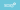#### MMP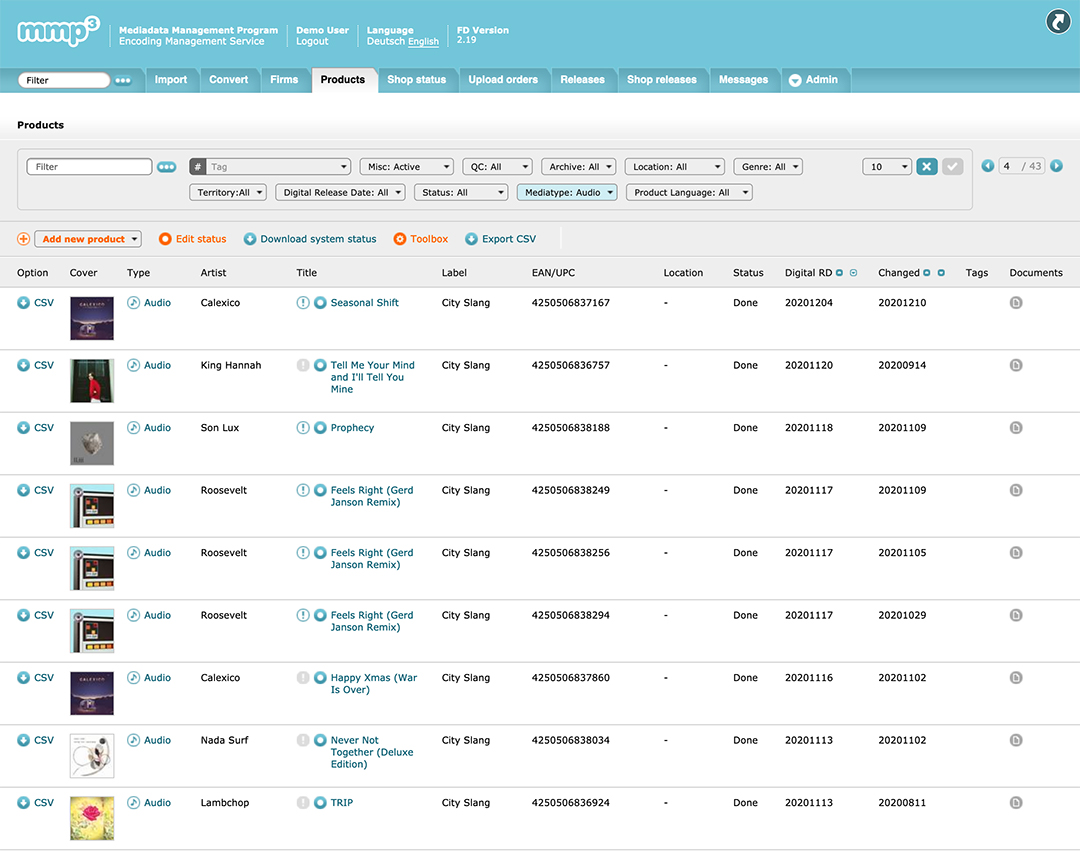###### Your digital command centre with us

Our exclusive, web-based logistics center, the Mediadata Management Program (MMP), allows you to administrate your data autonomously and in real-time. You keep total control over your materials, and enjoy access to the individual stages of the processing chain at all times, up to ultimately triggering the actual delivery to destinations.

You can:

• Edit every detail of your product meta data
• Navigate the rights, deals and delivery of your releases – from label-default right to individual details of your campaign
• Organize your co-contractors and labels.
• Optimize your team organization via our release-centered messaging system

The Mediadata Management Program (MMP) is the control center for your merchandise management with us.

### Artist Profiles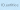#### ID.entities

##### Artist Profiles###### Full control of every artist profile in your catalogue

With ID.entities, you decide which artist profiles are addressed at Spotify and Apple with your products. You can query, adopt, correct, and merge provider-specific IDs and ensure your content appears exactly where it needs to be.
ID.entities gives you the best possible overview of your artists and products.

Always address the right artist identities on Spotify and Apple's services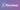#### Flasheet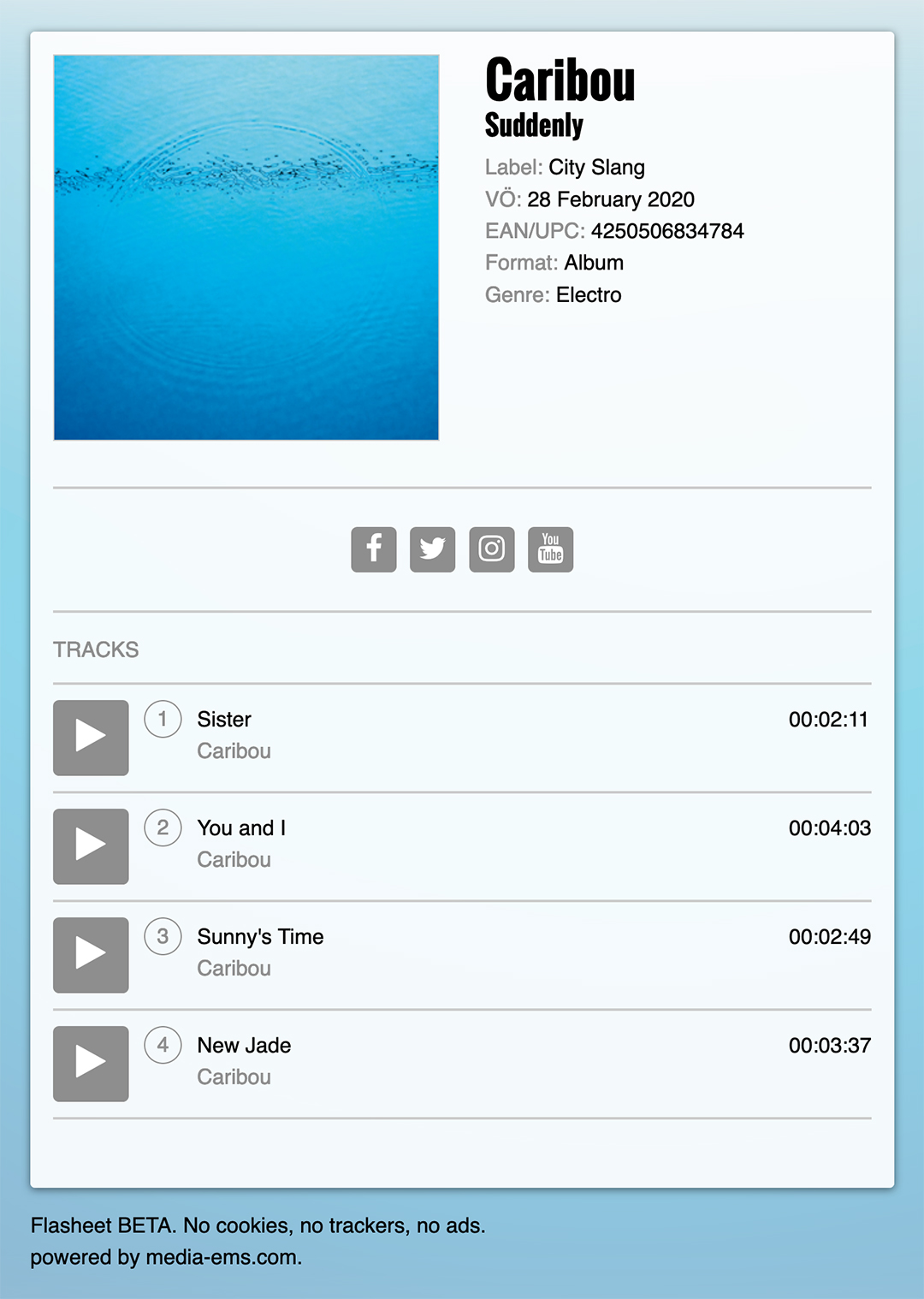###### A digital profile for your product

All the information about your artist, all collected in one place, just like on a good old flyer. Flasheet is its digital reincarnation, and includes all the most important facts and figures of your product, ready for your trade and media partners.

Digital One-Sheets for effectively pitching your products

### Know thy listener/s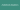#### Audiobook Analytics

##### Know thy listener/s###### All the data in one place

Together with ZEBRALUTION, we’ve developed our very own analytics tool for publishers, to give them detailed insights on their audiobook titles, authors and speakers. Our tool not only delivers topical updates on user numbers from across all relevant streaming services, but also provides information on target groups, device usage and genre trends. The findings from these data analyses can be used to assist publishers with the evaluation of their marketing campaigns, and even the planning of future activities and productions.

The usage numbers of your spoken content - at a glance, and actually telling you something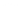#### SalesBoard###### Precise, up-to-date figures, delivered to you

Our in-house analytics tool “Salesboard” gives you the best possible overview of your sales and streaming figures. All the relevant numbers for your products and artists are sent to us by digital platforms from all across the world, and they’ll be waiting for you in our daily sales report.

We’re continuously working on expanding the scope of the reporting details to improve the valuation basis of your productions, and our goal is to deliver a clear, comprehensive and comprehendible overview of everything you need to know.

Sales figures and statistical evaluations, concise and clear.

### The analysis tool for music companies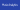#### Music Analytics

##### The analysis tool for music companies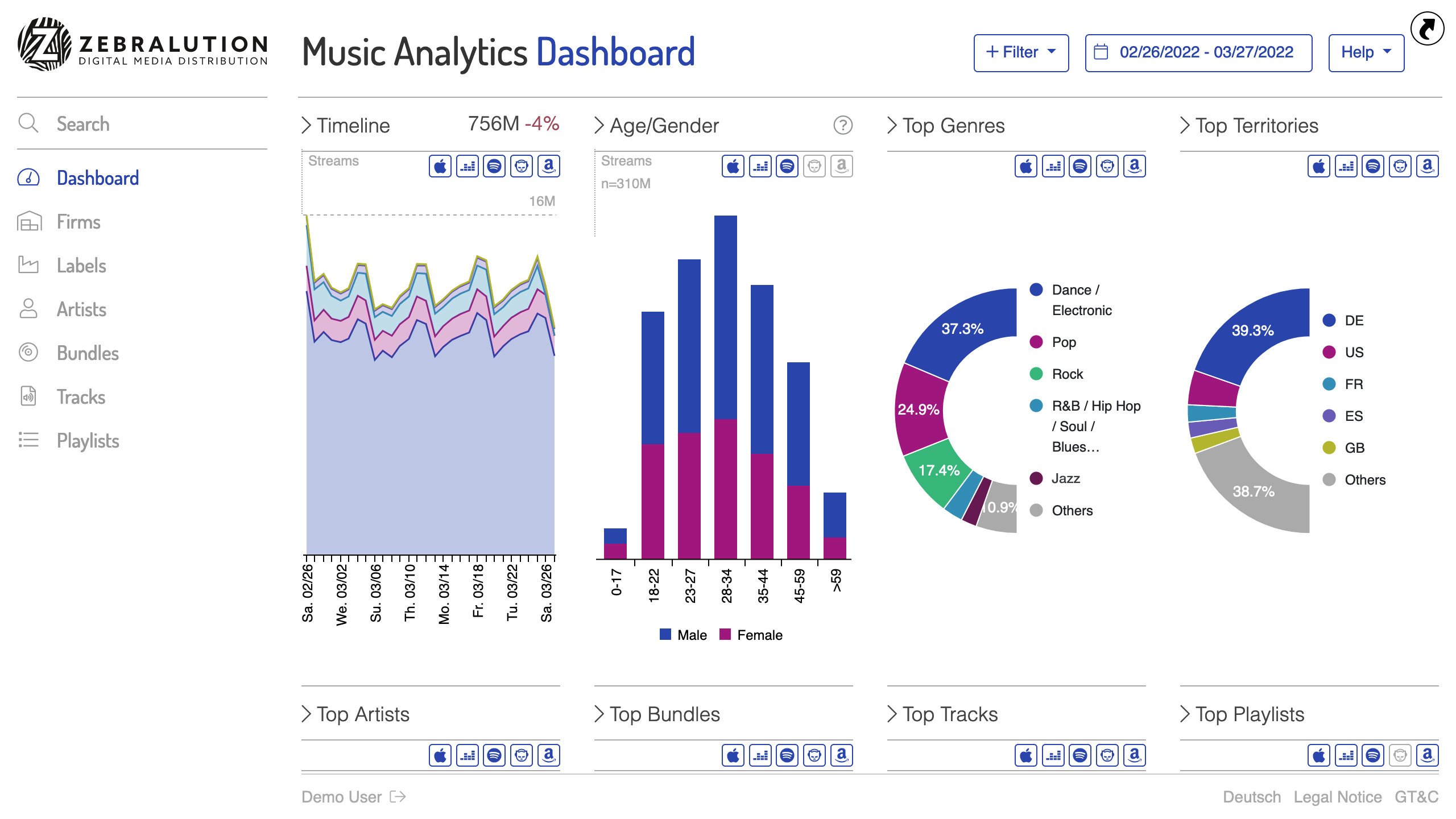###### Daily streaming performance in numbers and at a glance.

Music Analytics, developed in cooperation with Zebralution, helps our customers to improve the monitoring of the performance of their releases at the streaming providers. The tool also provides information about the target group, devices and most popular genres. These and other findings from the data analysis support labels in the evaluation of marketing campaigns, but also in the planning of future promotions and productions.

Daily streaming performance in numbers and at a glance.

## About usHuman-driven tech. Tech-driven humans.

### Pioneers, Coders, Musicians

A happy cruise on the Autobahn isn’t just about the vehicle, it’s also about the company.
For more than 20 years, our success has been down to more than our technical expertise. We also know your side of business, from our own experience. Because, in addition to professional engagement in dealing with media contents, a large part of our team is also active in the entertainment sector in their personal time; as artists, producers, label-managers and more. We all love music, good stories, and know all about the importance of the human factor. Because without it, even the best machine wouldn’t work.
We know about the value of your content and that’s why we treat it with a maximum of respect.

### Our values

EMS accepts nothing less than the highest security standards to protect your products and systems. Our servers are located in Germany and we rely on green energy, an obvious choice for reliability and long-term partnerships.

## Our TeamIn alphabetical order###### Alex Simon

Content Operations Manager###### Alexander Maiwald

System Operations Manager / DSP Relations###### Alina LohrmannAssistant to MDs & HR Manager###### Andreas Dutz

Content Manager Audiobook###### Bernd Kösling

Senior Developer###### Christian Kisseler

Content Operations Manager / Audiobook###### Christian Beermann

Content Operations Manager / Music###### Christoph Bornefeld-Ettmann

Managing Director###### Cynthia Meier

System Operations Manager###### Dominik Dwinger

Junior Developer###### Elmar Töller

Content Operations Manager / Music###### Erk Richter

System Operations Manager / Supply Chain###### Igor Dobric

Apprentice Developer###### Irmin Wiechmann

CTO###### Jan Küchler###### Joerg Nijmeijer

System Operations Manager / Product Owner MMP###### Julia Eichner

Content Operations Manager / Music###### Kevin Wagner

Content Operations Manager / Audiobook###### Klaus Kurtz

Senior Developer###### Levin Wiechmann

Creative Director###### Lukas Huettis

Team Leader Product Management & DevelopmentManaging Director###### Marius Melcher

Content Operations Manager / Audiobook###### Martin Schneider

Senior Manager Content Operations###### Norbert Töller

DeveloperSenior Developer

## Our NetworkTogether for your product

### Partnerships

Knowledge exchange is at the core of our work. Longtime partnerships with our customers and other important players in the market are a sign of trust for us. And, of course, we want you to benefit from our experience.

### Destinations

Sovereignty over all distribution contracts remains in your hands. We offer the logistical infrastructure, technological know-how and valuable relationships with more than 100 online retailers, streaming platforms, aggregators and other suppliers, from all over the world. Here’s a brief overview of the destinations we currently reach:

### The group

Since its inception in 2004, EMS has been working closely with Zebralution GmbH, constantly involved in the development of Europe's first digital distribution enterprise for many years. In 2019, we've transformed into our own division within the Zebralution family, working together even more tightly on new software solutions for the areas of digitally marketing music and spoken content in many forms.

## JobsWe are hiring

### What we offer

Flat hierarchies, family-friendly management and a trusting team atmosphere have always been important to us.

With us you are in the thick of the ongoing expansion and developments of the digital music and spoken contents markets.

### Vacancies

Fullstack Software Engineer (m/f/d)
Permanent position, full-time
Job posting

### Interested?

Then we look forward to your application to: